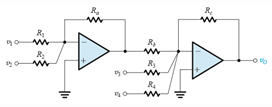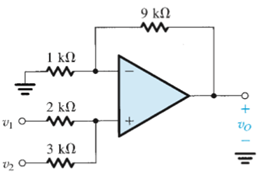Courses

Test: The Inverting Configuration & The Non Inverting Configuration

20 Questions MCQ Test Analog Electronics | Test: The Inverting Configuration & The Non Inverting Configuration

Description
Attempt Test: The Inverting Configuration & The Non Inverting Configuration | 20 questions in 20 minutes | Mock test for Electrical Engineering (EE) preparation | Free important questions MCQ to study Analog Electronics for Electrical Engineering (EE) Exam | Download free PDF with solutions
QUESTION: 1

When does a resistance provide a negative feedback to an amplifier?

Solution:

An op amp is said to have a negative feedback when a resistance is connected between the input and output terminals respectively.

QUESTION: 2

The effect of the inverting configuration is

Solution:

Inverting introduces a phase shift of 180o or it ‘inverts’ a peak.

QUESTION: 3

For an ideal negative feedback configuration which of the following is true?

Solution:

There is always a virtual short circuit in this type of case. There will be a virtual ground if and only if one of the terminals is grounded.

QUESTION: 4

The negative feedback causes

Solution:

Ideally the input terminals are at the same potential but in real practice there is a very small potential between the two terminals.

QUESTION: 5

The non-inverting closed loop configuration features a high resistance. Therefore in many cases unity gain follower called buffer amplifier is often used to

Solution:

Buffer amplifiers are required to connect a high resistance load to a low input resistance output.

QUESTION: 6

The advantage of a weighted summer operational amplifier is

Solution:

All of the mentioned are characteristics of a weighted summer operational amplifier over the traditional amplifier.

QUESTION: 7

The following is a circuit of weighted summer capable of summing coefficients of both sign. The expressions for the output voltage v0 isSolution:

The voltages are increased first by the left side of the portion and then are also magnified by the right side of the circuit. There are four inputs given out of which two are magnified twice and the other are magnified only once.

QUESTION: 8

You are provided with an ideal op amp and three 10kΩ resistors. Using series and parallel resistor combinations, how many different inverting-amplifier circuit topologies are possible?

Solution:

Consider series and parallel combination of the resistances provided and arrange then in the feedback region and as output resistance.

QUESTION: 9

The loop gain for an ideal operational amplifier with R1 = 10kΩ and R2(negative feedback) = 1MΩ is

Solution:

Loop gain in this case is given by 20 log (1000000/10000).

QUESTION: 10

In an inverting op-amp circuit for which the gain is −4 V/V and the total resistance used is 100 kΩ. Then the value of R1 and R2 (negative feedback)

Solution:

Solve R1 + R2 = 100
R2/R1 = 4 for R1 and R2 respectively.

QUESTION: 11

In the non-inverting configuration of operational amplifier

Solution:

Non inverting configuration requires a power source connected to the power source.

QUESTION: 12

For ideal non-inverting operational amplifier

Solution:

It is an ideal characteristic of the non-inverting op amp.

QUESTION: 13

For an ideal non-inverting operational amplifier having finite gain (A), the ratio of output voltage (v0) to input voltage (vi) is (given R2 is the feedback resistance)

Solution:

It is a standard mathematical expression.

QUESTION: 14

The gain for an ideal non-inverting operational amplifier is (given R2 is the feedback resistance)

Solution:

It is a standard mathematical expression.

QUESTION: 15

While performing an experiment to determine the gain for an ideal operational amplifier having finite gain, a student mistakenly used the equation 1 + R2/R1 where R2 is the feedback resistance. What is the percentage error in his result? Given A is the finite voltage gain of the ideal amplifier used.

Solution:

The correct formula is (1+R2/R1)/(1+((1+R2/R1)/A)).

QUESTION: 16

The finite voltage gain of a non-inverting operational amplifier is A and the resistance used is R1 and R2 in which R2 is the feedback resistance. Under what conditions it can one use the expression 1 + R2/R1 to determine the gain of the amplifier?

Solution:

The formula is valid for the ideal case in which the value of A is infinite, practically it should be very large when compared to R2/R1 .

QUESTION: 17

Which of the following is not true for a voltage follower amplifier?

Solution:

All the statements are false.

QUESTION: 18

For designing a non-inverting amplifier with a gain of 2 at the maximum output voltage of 10 V and the current in the voltage divider is to be 10 μA the resistance required are R1 and R2where R2 is used to provide negative feedback. Then

Solution:

1 + R2/R1 = 2 and 10/(R1+R2) = 10 μA. Solve for R1 and R2.

QUESTION: 19

It is required to connect a transducer having an open-circuit voltage of 1 V and a source resistance of 1 MΩ to a load of 1-kΩ resistance. Find the load voltage if the connection is done

Solution:

When a unity gain follower is uses then input signal is equal to output signal. When connected directly, output signal is given by 1 X 1kΩ/1MΩ or 1mV.

QUESTION: 20

Consider the figure given below. If the resistance R1 is disconnected from the ground and connected to a third power source v3, then expression for the value of v0 isSolution:

When a third power source is connected to the resistance of 1kΩ, then also the potential between the two input terminals of op amps remains the same. Using this fact the expression c is obtained.Use Code STAYHOME200 and get INR 200 additional OFF Use Coupon Code

Track your progress, build streaks, highlight & save important lessons and more!

Similar ContentRelated tests